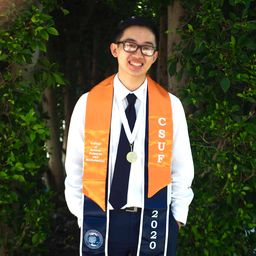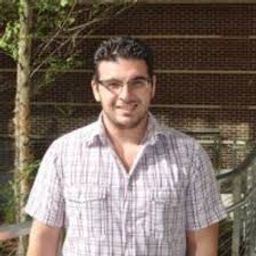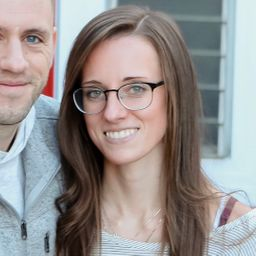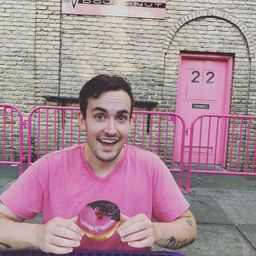💬 👋 We’re always here. Join our Discord to connect with other students 24/7, any time, night or day.Join Here!

# Biology 2015

## EducatorsJA+ 4 more educators

### Problem 1

For questions 1–4, match each stage of the cell cycle to its correct description.
Key:
$\begin{array}{ll}{\text { a. } G_{1} \text { stage }} & {\text { b. S stage }} \\ {\text { c. } G_{2} \text { stage }} & {\text { d. } M(\text { mitotic }) \text { stage }}\end{array}$

At the end of this stage, each chromosome consists of two attached chromatids.Eric T.

### Problem 2

For questions , match each stage of the cell cycle to its correct description.
Key:
$\begin{array}{ll}{\text { a. } G_{1} \text { stage }} & {\text { b. S stage }} \\ {\text { c. } G_{2} \text { stage }} & {\text { d. } M(\text { mitotic }) \text { stage }}\end{array}$

During this stage, daughter chromosomes are distributed to two daughter nuclei.Nalvi D.

### Problem 3

For questions , match each stage of the cell cycle to its correct description.
Key:
$\begin{array}{ll}{\text { a. } G_{1} \text { stage }} & {\text { b. S stage }} \\ {\text { c. } G_{2} \text { stage }} & {\text { d. } M(\text { mitotic }) \text { stage }}\end{array}$

The cell doubles its organelles and accumulates the materials needed for DNA synthesis.

JA
Jamelia A.

### Problem 4

For questions , match each stage of the cell cycle to its correct description.
Key:
$\begin{array}{ll}{\text { a. } G_{1} \text { stage }} & {\text { b. S stage }} \\ {\text { c. } G_{2} \text { stage }} & {\text { d. } M(\text { mitotic }) \text { stage }}\end{array}$

The cell synthesizes the proteins needed for cell division.Nalvi D.

### Problem 5

Which is not true of the cell cycle?

a. The cell cycle is controlled by internal/external signals.
b. Cyclin is a signaling molcule that increases and decreases as the cycle continues.
c. DNA damage can stop the cell cycle at the G1 checkpoint.
d. Apoptosis occurs frequently during the cell cycle.Alexander C.

### Problem 6

The diploid number of chromosomes

a. is the 2n number.
b. is in a parent cell and therefore in the two daughter cells following mitosis.
c. varies according to the particular organism.
d. is present in most somatic cells.
e. All of these are correct.Nalvi D.

### Problem 7

The form of DNA that contains genes that are actively being transcribed is called

a. histones.
b. telomeres.
c. heterochromatin.
d. euchromatin.Alyssa M.

### Problem 8

Histones are involved in

a. regulating the checkpoints of the cell cycle.
b. lengthening the ends of the telomeres.
c. compacting the DNA molecule.
d. cytokinesis.Nalvi D.

### Problem 9

At the metaphase plate during metaphase of mitosis, there are

a. single chromosomes.
b. duplicated chromosomes.
c. G1 stage chromosomes.
d. always 23 chromosomesBryan L.

### Problem 10

During which mitotic phases are duplicated chromosomes present?

a. all but telophase
b. prophase and anaphase
c. all but anaphase and telophase
d. only during metaphase at the metaphase plate
e. Both a and b are correct.Nalvi D.

### Problem 11

Which of these is paired incorrectly?

a. prometaphase - the kinetochores become attached to spindle fibers
b. anaphase- daughter chromosomes migrate toward spindle poles
c. prophase - the nucleolus disappears and the nuclear envelope disintegrates
d. metaphase - the chromosomes are aligned in the metaphase plate
e. telophase - a resting phase between cell division cycles

KA
Kemi A.

### Problem 12

Which of the following is not characteristic of cancer cells?

a. Cancer cells often undergo angiogenesis.
b. Cancer cells tend to be nonspecialized.
c. Cancer cells undergo apoptosis.
d. Cancer cells often have abnormal nuclei.
e. Cancer cells can metastasize.Nalvi D.

### Problem 13

Which of the following statements is true?

a. Proto-oncogenes cause a loss of control of the cell cycle.
b. The products of oncogenes may inhibit the cell cycle.
c. Tumor suppressor gene products inhibit the cell cycle.
d. A mutation in a tumor suppressor gene may inhibit the cell cycle.Bryan L.

### Problem 14

In contrast to a eukaryotic chromosome, a prokaryotic chromosome

a. is shorter and fatter.
b. has a single loop of DNA.
c. never replicates.
d. contains many histones.
e. All of these are correct.Nalvi D.